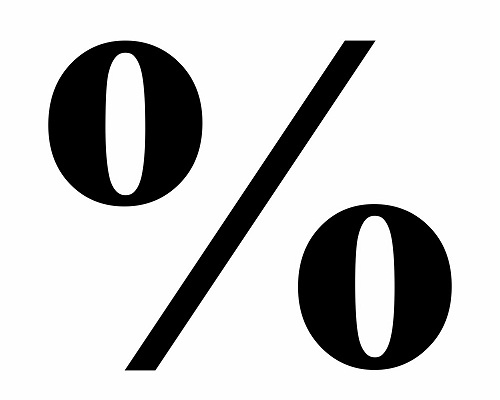# Percentage Calculator

Percentage means out of 100, which means divide by 100. Simple Percentage calculator online tool to calculate the original percentage of value from an amount of total price. Also, determine what is the increase percentage or decrease percentage in a value.

The term "percent" is derived from the Latin per centum, meaning hundred or by the hundred. The percent value is computed by multiplying the numeric value of the ratio by 100.

## Definition and Facts About Percentage

The concept of percentage is an extension of the material we have covered about fractions and ratio. To allow easy comparisons of fractions we need to use the same denominator. As such, percentages use 100 as the denominator. In other words, the ‘whole’ is divided into 100 equal parts. The word “per cent” means per 100. Therefore 27% is 27 / 100 .

To use percentage in a calculation, the simple mathematical procedure is modelled below where we write the percentage as a fraction with a denominator of 100. For example: 25% is 25 / 100, thus to calculate 25%of 40, we could approach it as (25 / 100) × (40 / 1)=10

Percentages are most commonly used to compare parts of an original. For instance, the phrase 30% off sale! indicates that whatever the original price, the new price will be 30% less.

However, percentages are rarely as simple as 23% of 60. Percentages are more commonly used as part of a more complex question. Often the question is, “How much is left?” or “How much was the original?”### Spreading Knowledge Across the World

USA - United States of America  Canada  United Kingdom  Australia  New Zealand  South America  Brazil  Portugal  Netherland  South Africa  Ethiopia  Zambia  Singapore  Malaysia  India  China  UAE - Saudi Arabia  Qatar  Oman  Kuwait  Bahrain  Dubai  Israil  England  Scotland  Norway  Ireland  Denmark  France  Spain  Poland  and  many more....